×#### Thank you for registering.

One of our academic counsellors will contact you within 1 working day.

Click to Chat

1800-1023-196

+91-120-4616500

CART 0

• 0

MY CART (5)

Use Coupon: CART20 and get 20% off on all online Study Material

ITEM
DETAILS
MRP
DISCOUNT
FINAL PRICE
Total Price: Rs.

There are no items in this cart.
Continue Shopping• Complete JEE Main/Advanced Course and Test Series
• OFFERED PRICE: Rs. 15,900
• View Details

```Chapter 15: Areas of Parallelograms And Triangles Exercise – 15.3

Question: 1

In figure, compute the area of quadrilateral ABCD.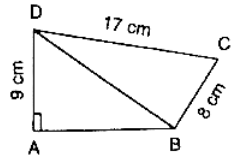Solution:

Given:

DC = 17 cm, AD = 9 cm and BC = 8 cm

In ΔBCD we have

CD2 = BD2 + BC2

⇒ 172 = BD2 + 82

⇒ BD2 = 289 − 64

⇒ BD = 15

In ΔABD we have

⇒ 152 = AB2 + 92

⇒ AB2 = 225 − 81 = 144

⇒ AB = 12

ar(quad ABCD) = ar(ΔABD) + ar(ΔBCD)

ar(quad ABCD) = 1/2(12 × 9) + 1/2(8 × 17) = 54 + 68 = 122 cm2

ar(quad ABCD) = 1/2(12 × 9) + 1/2(8 × 15) = 54 + 60 = 114 cm2

Question: 2

In figure, PQRS is a square and T and U are, respectively, the midpoints of PS and QR. Find the area of ΔOTS if PQ = 8 cm.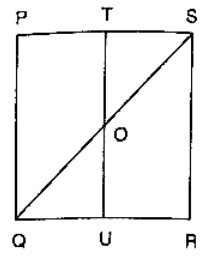Solution:

From the figure,

T and U are mid points of PS and QR respectively

∴ TU ∥ PQ

⇒ TO ∥ PQ

Thus, in ΔPQS, T   is the midpoint of PS and TO ∥ PQ

∴ TO = (1/2) PQ = 4 cm

Also, TS = (1/2) PS = 4 cm

∴ ar(ΔOTS) = (1/2)(TO × TS) = (1/2)(4 × 4)cm2 = 8 cm2

Question: 3

Compute the area of trapezium PQRS in figureSolution:

We have,

ar(trap. PQRS) = ar(rect. PSRT) + ar(ΔQRT)

⇒ ar(trap. PQRS) = PT × RT + 1/2(QT × RT)

= 8 × RT + 1/2(8 × RT) = 12 × RT

In ΔQRT, we have

QR2 = QT2 + RT2

⇒ RT2 = QR2 − QT2

⇒ RT2 = 172 − 82 = 225

⇒ RT = 15

Hence, Area of trapezium = 12 × 15 cm2 = 180 cm2

Question: 4

In figure, ∠AOB = 90°, AC = BC, OA = 12 cm and OC = 6.5 cm. Find the area of ΔAOB.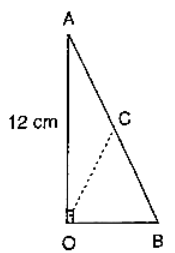Solution:

Since, the midpoint of the hypotenuse of a right triangle is equidistant from the vertices

∴ CA = CB = OC

⇒ CA = CB = 6.5 cm

⇒ AB = 13 cm

In right angled triangle OAB, we have

AB2 = OB2 + OA2

⇒ 132 = OB2 + 122

⇒ OB2 = 132 − 122 = 169 − 144 = 25

⇒ OB = 5

∴ ar(ΔAOB) = (1/2)(12 × 5) = 30 cm2

Question: 5

In figure, ABCD is a trapezium in which AB = 7 cm, AD = BC = 5 cm, DC = x cm, and distance between AB and DC is 4 cm. Find the value of x and area of trapezium ABCD.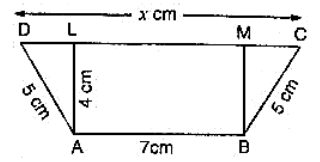Solution:

Draw AL ⊥ DC, BM ⊥ DC then,

AL = BM = 4 cm and LM = 7 cm.

⇒ 25 = 16 + DL2

⇒ DL = 3 cm

Similarly,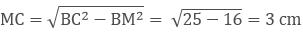∴ x = CD = CM + ML + LD = (3 + 7 + 3) cm = 13 cm

ar(trap. ABCD) = 1/2(AB + CD) × AL = 1/2(7 + 13) × 4 cm2 = 40 cm2

Question: 6

In figure, OCDE is a rectangle inscribed in a quadrant of a circle of radius 10 cm. If OE = 2√5 cm, find the area of the rectangle.Solution:

Given OD = 10 cm and OE = 2√5cm

By using Pythagoras theorem

∴ OD2 = OE2 + DE2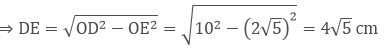∴ Area of rectangle OCDE = OE × DE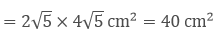Question: 7

In figure, ABCD is a trapezium in which AB ∥ DC. Prove that ar(ΔAOD) = ar(ΔBOC)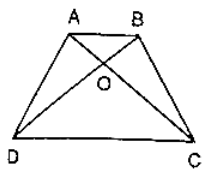Solution:

Given:  ABCD is a trapezium in which AB ∥ DC

To prove: ar(ΔAOD) = ar(ΔBOC)

Proof: Since, ΔADC and ΔBDC are on the same base DC and between same parallels AB and DC

⇒ ar(ΔAOD) + ar(ΔDOC) = ar(ΔBOC) + ar(ΔDOC)

⇒ ar(ΔAOD) = ar(ΔBOC)

Question: 8

In figure, ABCD, ABFE and CDEF are parallelograms. Prove that ar(ΔADE) = ar(ΔBCF).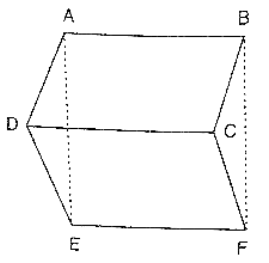Solution:

Given that

ABCD is parallelogram ⇒ AD = BC

CDEF is parallelogram ⇒ DE = CF

ABFE is parallelogram ⇒ AE = BF

Thus, in Δs ADF and BCF, we have

AD = BC, DE = CF and AE = BF

So, by SSS criterion of congruence, we have

Question: 9

Diagonals AC and BD of a quadrilateral ABCD intersect each other at P. Show that: ar(ΔAPB) × ar(ΔCPD) = ar(ΔAPD) × ar(ΔBPC).

Solution:

Construction: - Draw BQ ⊥ AC and DR ⊥ AC

Proof:-

L.H.S

= ar(ΔAPB) × ar(ΔCDP)

= (1/2) [(AP × BQ)] × (1/2 × PC × DR)

= (1/2 × PC × BQ) × (1/2 × AP × DR)

= ar(ΔAPD) × ar(ΔBPC).

= R.H.S

Hence proved.

Question: 10

In figure, ABC and ABD are two triangles on the base AB. If line segment CD is bisected by AB at O, show that ar(ΔABC) = ar(ΔABD).Solution:

Given that CD is bisected by AB at O

To prove: ar(ΔABC) = ar(ΔABD).

Construction: Draw CP ⊥ AB and DQ ⊥ AB.

Proof:

ar(ΔABC) = 1/2 × AB × CP⋅⋅⋅⋅⋅ (1)

ar(ΔABD) = 1/2 × AB × DQ ⋅⋅⋅⋅ (2)

In ΔCPO and ΔDQO

∠CPO = ∠DQO  [Each 90°]

Given that, CO = OD

∠COP = ∠DOQ  [Vertically opposite angles are equal]

Then, ΔCPO ≅ ΔDQO  [By AAS condition]

∴ CP = DQ (3)   [C.P.C.T]

Compare equation (1), (2) and (3)

∴ ar(ΔABC) = ar(ΔABD).

Question: 11

If P is any point in the interior of a parallelogram ABCD, then prove that area of the triangle APB is less than half the area of parallelogram.

Solution:

Draw DN ⊥ AB and PM ⊥ AB

Now,

ar (∥gm ABCD) = AB × DN, ar(ΔAPB) = (1/2) (AB × PM)

Now, PM < DN

⇒ AB × PM < AB × DN

⇒ (1/2)(AB × PM) < (1/2)(AB × DN)

⇒ ar(ΔAPB) < 1/2 ar(∥ gm ABCD)

Question: 12

If AD is a median of a triangle ABC, then prove that triangles ADB and ADC are equal in area. If G is the mid-point of the median AD, prove that ar(ΔBGC) = 2ar(ΔAGC).

Solution:

Draw AM ⊥ BC

Since, AD is the median of ΔABC

∴ BD = DC

⇒ BD = AM = DC × AM

⇒ (1/2)(BD × AM) = (1/2)(DC × AM)

⇒ ar(ΔABD) = ar(ΔACD) ⋅⋅⋅⋅⋅⋅ (1)

In ΔBGC, GD is the median

⇒ ar(ΔBGD) = ar(ΔCGD) ⋅⋅⋅⋅⋅⋅ (2)

In ΔACD, CG is the median

⇒ ar(ΔAGC) = ar(ΔCGD) ⋅⋅⋅⋅⋅ (3)

From (2) and (3) we have,

ar(ΔBGD) = ar(ΔAGC)

But, ar(ΔBGC) = 2ar(ΔBGD)

⇒ ar(ΔBGC) = 2ar(ΔAGC)

Question: 13

A point D is taken on the side BC of a ΔABC, such that BD = 2DC. Prove that ar(ΔABD) = 2ar(ΔADC).

Solution:

Given that,

In ΔABC, BD = 2DC

Construction:

Take a point E on BD such that BE = ED

Proof: Since, BE = ED and BD = 2 DC

Then, BE = ED = DC

We know that median of triangle divides it into two equal triangles.

∴ In ΔABD, AE is the median.

Then, ar(ΔABD) = 2ar(ΔAED) ⋅⋅⋅⋅ (1)

In ΔAEC, AD is the median.

Then, ar(ΔAED) = 2ar(ΔADC) ⋅⋅⋅ (2)

Compare equation 1 and 2

Question: 14

ABCD is a parallelogram whose diagonals intersect at O .If P is any point on BO, prove that:

(ii) ar(ΔABP) = 2ar(ΔCBP).

Solution:

Given that ABCD is the parallelogram

To Prove:

(ii) ar(ΔABP) = 2ar(ΔCBP).

Proof:

we know that diagonals of parallelogram bisect each other

∴  AO = OC and BO = OD

(i) In ΔDAC, since DO is a median.

(ii) In ΔBAC, since BO is a median.

Then ar(ΔBAO) = ar(ΔBCO) ⋅⋅⋅⋅ (1)

In ΔPAC, since PO is a median.

Then ar(ΔPAO) = ar(ΔPCO) ⋅⋅⋅⋅ (2)

Subtract equation 2 from 1.

⇒ ar(ΔBAO) − ar(ΔPAO) = ar(ΔBCO) − ar(ΔPCO)

⇒ ar(ΔABP) = 2ar(ΔCBP).

Question: 15

ABCD is a parallelogram in which BC is produced to E such that CE = BC. AE intersects CD at F.

(i) Prove that ar(ΔADF) = ar(ΔECF).

(ii) If the area of ΔDFB = 3 cm2, find the area of ∥ gm ABCD.

Solution:

In triangles ADF and ECF, we have

And ∠DFA = ∠CFA   [Vertically opposite angles]

So, by AAS congruence criterion, we have

⇒ ar(ΔADF) = ar(ΔECF) and DF = CF .

Now, DF = CF

⇒ BF is a median in Δ BCD.

⇒ ar(ΔBCD) = 2ar(ΔBDF)

⇒ ar(ΔBCD) = 2 × 3 cm2 = 6 cm2

Hence, area of a parallelogram = 2ar(ΔBCD) = 2 × 6 cm2 = 12 cm2

Question: 16

ABCD is a parallelogram whose diagonals AC and BD intersect at O. A line through O intersects AB at P and DC at Q. Prove that ar(ΔPOA) = ar(ΔQOC).

Solution:

In triangles POA and QOC, we have

∠AOP = ∠COQ

AO = OC

∠PAC = ∠QCA

So, by ASA congruence criterion, we have

ΔPOA ≅ ΔQOC

⇒ ar(ΔPOA) = ar(ΔQOC).

Question: 17

ABCD is a parallelogram. E is a point on BA such that BE = 2EA and F is point on DC such that DF = 2FC. Prove that AECF is a parallelogram whose area is one third of the area of parallelogram ABCD.

Solution:

Draw FG ⊥ AB

We have,

BE = 2 EA and DF = 2FC

⇒ AB - AE = 2 AE and DC - FC = 2 FC

⇒ AB = 3 AE and DC = 3 FC

⇒ AE = (1/3) AB and FC = (1/3)DC ⋅⋅⋅⋅ (1)

But AB = DC

Then, AE = FC     [opposite sides of ∥gm]

Thus, AE = FC and AE ∥ FC

Then, AECF is a parallelogram

Now, area of parallelogram AECF = AE × FG

⇒ ar(∥gm AECF) = 1/3 AB × FG       from(1)

⇒ 3ar (∥gm AECF) = AB × FG            ⋅⋅⋅ (2)

And ar(∥gm ABCD) = AB × FG ⋅⋅⋅ (3)

Compare equation 2 and 3

⇒ 3ar(∥gm AECF) = ar(∥gm ABCD)

⇒ ar(∥gm AECF) = 1/3 ar(∥gm ABCD)

Question: 18

In a triangle ABC, P and Q are respectively the mid points of AB and BC and R is the midpoint of AP. Prove that:

(i) ar(ΔPBQ) = ar(ΔARC).

(ii) ar(ΔPRQ) = 1/2 ar(ΔARC).

(iii) ar(ΔRQC) = 3/8 ar(ΔABC).

Solution:

We know that each median of a triangle divides it into two triangles of equal area.

(i) Since CR is the median of ΔCAP

∴ ar(ΔCRA) = (1/2) ar(ΔCAP)   ⋅⋅⋅⋅⋅ (1)

Also, CP is the median of a Δ CAB

∴ ar(ΔCAP) = ar(ΔCPB)  ⋅⋅⋅⋅ (2)

From 1 and 2, we get

∴ ar(ΔARC) = (1/2) ar(ΔCPB)  ⋅⋅⋅⋅⋅  (3)

PQ is the median of a ΔPBC

∴ ar(ΔCPB) = 2ar(ΔPBQ)   ⋅⋅⋅ (4)

From 3 and 4, we get

∴ ar(ΔARC) = ar(ΔPBQ)   ⋅⋅⋅⋅ (5)

(ii) Since QP and QR medians of triangles QAB and QAP respectively

∴ ar(ΔQAP) = ar(ΔQBP)   ⋅⋅⋅⋅  (6)

And ar(ΔQAP) = 2ar(ΔQRP) ⋅⋅⋅⋅ (7)

From 6 and 7, we get

ar(ΔPRQ) = (1/2) ar(ΔPBQ) ⋅⋅⋅⋅⋅ (8)

From 5 and 8, we get

ar(ΔPRQ) = (1/2) ar(ΔARC)

(iii) Since, LR is a median of ΔCAP

= 1/2 × (1/2) ar(ΔABC)

= (1/4) ar(ΔABC)

Since RQ is the median of ΔRBC.

∴ ar(ΔRQC) = (1/2) ar(ΔRBC)

= (1/2) {ar(ΔABC) − ar(ΔARC)}

= (1/2) {ar(ΔABC) – (1/4) ar(ΔABC)}

= (3/8) ar(ΔABC)

Question: 19

ABCD is a parallelogram. G is a point on AB such that AG = 2GB and E is point on DC such that CE = 2DE and F is the point of BC such that BF = 2FC. Prove that:

(ii) ar(ΔEGB) = (1/6) ar(ABCD)

(iii) ar(ΔEFC) = (1/2) ar(ΔEBF)

(iv) ar(ΔEGB) = 3/2 × ar(ΔEFC)

(v) Find what portion of the area of parallelogram is the area of ΔEFG.

Solution:

Given: ABCD is a parallelogram

AG = 2 GB, CE = 2 DE and BF = 2 FC

To prove:

(ii) ar(ΔEGB) = (1/6) ar(ABCD).

(iii) ar(ΔEFC) = (1/2) ar(ΔEBF).

(iv) ar(ΔEGB) = (3/2) × ar(ΔEFC)

(v) Find what portion of the area of parallelogram is the area of ΔEFG.

Construction: Draw a parallel line to AB through point F and a perpendicular line to AB from C

Proof:(i) Since ABCD is a parallelogram

So, AB = CD and AD = BC

Consider the two trapezium s ADEG and GBCE

Since AB = DC, EC = 2DE, AG = 2GB

⇒ ED = (1/3) CD = (1/3) AB and EC = (2/3) CD = (2/3) AB

⇒ AG = (2/3) AB and BG = (1/3) AB

So, DE + AG = (1/3) AB + (2/3) AB = AB and EC + BG = (2/3) AB + (1/3) AB = AB

Since the two trapezium ADEG and GBCE have same height and their sum of two parallel sides are equal

Since(ii) Since we know from above that

BG = (1/2) AB So

ar(ΔEGB) = (1/2) × GB × Height

ar(ΔEGB) = (1/2) × (1/3) × AB × Height

ar(ΔEGB) = (1/6) × AB × Height

ar(ΔEGB) = (1/6) ar(ABCD).

(iii) Since height if triangle EFC and EBF are equal. So

ar(ΔEFC) = (1/2) × FC × Height

ar(ΔEFC) = (1/2) × (1/2) × FB × Height

ar(ΔEFC) = (1/2) ar(EBF)

Hence, ar(ΔEFC) = (1/2) ar(ΔEBF).

(iv) Consider the trapezium in which

ar(EGBC) = ar(ΔEGB) + ar(ΔEBF) + ar(ΔEFC)

⇒ (1/2) ar(ABCD) = (1/6) ar(ABCD) + 2ar(ΔEFC) + ar(ΔEFC)

⇒ (1/3) ar(ABCD) = 3 ar(ΔEFC)

⇒ ar(ΔEFC) = (1/9) ar(ABCD)

Now from (ii) part we have

ar(ΔEGB) = (1/6) ar(ΔEFC)

ar(ΔEGB) = (3/2) × (1/9) ar(ABCD)

ar(ΔEGB) = (3/2) ar(ΔEFC)

∴ ar(ΔEGB) = (3/2) ar(ΔEFC)

(v) In the figure it is given that FB = 2CF.

Let CF = x and FB = 2x.

Now consider the two triangles CFI and CBH which are similar triangle.

So by the property of similar triangle CI = k and IH = 2k

Now consider the triangle EGF in which

ar(ΔEFG) = ar(ΔESF) + ar(ΔSGF)

ar(ΔEFG) = (1/2) SF × k + (1/2) SF × 2k

ar(ΔEFG) = (3/2) SF × k   ⋅⋅⋅ (i)

Now,

ar(ΔEGBC) = ar(SGBF) + ar(ESFC)

ar(ΔEGBC) = (1/2)(SF + GB) × 2k + (1/2)(SF + EC) × k

ar(ΔEGBC) = (3/2) k × SF + (GB + (1/2)EC) × k

ar(ΔEGBC) = (3/2) k × SF + (1/3 AB + (1/2) × (2/3) AB) × k

(1/2) ar(ΔABCD) = (3/2) k × SF + (2/3) AB × k

⇒ ar(ΔABCD) = 3k × SF + (4/3) AB × k       [Multiply both sides by 2]

⇒ ar(ΔABCD) = 3k × SF + 4/9 ar(ABCD)

⇒ k × SF = (5/27 )ar(ABCD) ⋅⋅⋅⋅  (2)

From 1 and 2 we have,

ar(ΔEFG) = (3/2) × (5/27) ar(ABCD)

ar(ΔEFG) = (5/18) ar(ABCD)

Question: 20

In figure, CD ∥ AE and CY ∥ BA.

(i) Name a triangle equal in area of ΔCBX

(ii) Prove that ar(ΔZDE) = ar(ΔCZA)

(iii) Prove that ar(BCZY) = ar(ΔEDZ)Solution:

Since, triangle BCA and triangle BYA are on the same base BA and between same parallel s BA and CY.

Then ar(ΔBCA) = ar(ΔBYA)

⇒ ar(ΔCBX) + ar(ΔBXA) = ar(ΔBXA) + ar(ΔAXY)

⇒ ar(ΔCBX) = ar(ΔAXY) ⋅⋅⋅⋅ (1)

Since, triangles ACE and ADE are on the same base AE and between same parallels CD and AE

ar(ΔCZA) + ar(ΔAZE) = ar(ΔAZE) + ar(ΔDZE)

ar(ΔCZA) = ar(ΔDZE) ⋅⋅⋅ (2)

Adding ar(ΔCYG) on both sides , we get

⇒ ar(ΔCBX) + ar(ΔCYZ) = ar(ΔCAY) + ar(ΔCYZ)

⇒ ar(BCZY) = ar(ΔCZA) ⋅⋅⋅ (3)

Compare equation 2 and 3

⇒ ar(BCZY) = ar(ΔDZE)

Question: 21

In figure, PSDA is a parallelogram in which PQ = QR = RS and AP ∥ BQ ∥ CR. Prove that ar (ΔPQE) = ar(ΔCFD).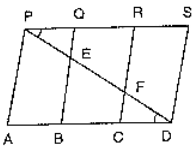Solution:

Given that PSDA is a parallelogram

Since, AP ∥ BQ ∥ CR ∥ DS and AD ∥ PS

Therefore, PQ = CD       (equ. 1)

In triangle BED, C is the midpoint of BD and CF ∥ BE

Therefore, F is the midpoint of ED

⇒ EF = PE

Similarly,

EF = PE

Therefore, PE = FD             (equ. 2)

In triangles PQE and CFD, we have

PE = FD

Therefore, ∠ EPQ = ∠FDC     [Alternate angles]

So, by SAS criterion, we have

ΔPQE ≅ ΔDCF

⇒ ar(ΔPQE) = ar(ΔDCF)

Question: 22

In figure, ABCD is a trapezium in which AB || DC and DC = 40 cm and AB = 60 cm .If X and Y are, respectively, the mid points of AD and BC, prove that:

(i) XY = 50 cm

(ii) DCYX is a trapezium

(iii) ar(trap. DCYX) = (9/11) ar(XYBA).Solution:

(i) Join DY and produce it to meet AB produced at P.

In triangles BYP and CYD we have,

∠BYP = ∠ CYD          [Vertically opposite angles]

∠DCY = ∠ PBY           [Since, DC ∥ AP]

And BY = CY

So, by ASA congruence criterion, we have

(ΔBYP) ≅ (ΔCYD)

⇒ DY = Yp and DC = BP

⇒ Y is the midpoint of DP

Also, x is the midpoint of AD

Therefore, XY ∥ AP and XY ∥ (1/2) AP

⇒ XY = (1/2) (AB + BP)

⇒ XY = (1/2) (AB + DC)

⇒ XY = (1/2) (60 + 40)

= 50 cm

(ii) We have, XY ∥ AP

⇒ XY ∥ AB and AB ∥ DC

⇒ XY ∥ DC

⇒ DCYX is a trapezium

(iii) Since x and y are the mid points of Ad and BC respectively.

Therefore, trapezium DCYX and ABYX are of the same height say h cm

Now,

ar(trap. DCXY) = (1/2)(DC + XY) × h

⇒ ar(trap. DCXY) = (1/2) (50 + 40) × h cm2 = 45 h cm2

⇒ ar(trap. ABYX) = (1/2)(AB + XY) × h

⇒ ar(trap. ABYX) = (1/2)(60 + 50) × h cm2 = 55h cm2

ar(trap. DCYX) ar(trap. ABYX) = 45h/55h = 9/11

⇒ ar(trap. DCYX) = 9/11 ar(trap. ABYX)

Question: 23

In figure ABC and BDE are two equilateral triangles such that D is the midpoint of BC. AE intersects BC in F. Prove that: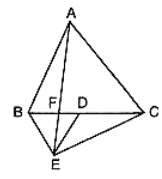(i) ar(ΔBDE) = (1/4) ar(ΔABC)

(ii) ar(ΔBDE) = (1/2) ar(ΔBAE)

(iii) ar(ΔBFE) = ar(ΔAFD)

(iv) ar(ΔABC) = 2 ar(ΔBEC)

(v) ar(ΔFED) = 1/8 ar(ΔAFC)

(vi) ar(ΔBFE) = 2 ar(ΔEFD)

Solution:

Given that ABC and BDE are two equilateral triangles.

Let AB = BC = CA = x. Then, BD = x/2 = DE = BE

(i) We have,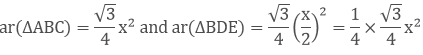Therefore, ar(ΔBDE) = 1/4 ar(ΔABC).

(ii) It is given that triangles, ABC and BED are equilateral triangles

∠ ACB = ∠DBE = 60°

⇒ BE ∥ AC (Since, Alternative angles are equal)

Trinagles BAF and BEC are on the same base BE and between same parallels BF and AC.

Therefore, ar(ΔBAE) = ar(ΔBEC)

⇒ ar(ΔBAE) = 2ar(ΔBDE)      [Since , ED is a median of triangle EBC; ar(ΔBEC) = 2ar(ΔBDE) ]

∴ ar(ΔBDE) = (1/2) ar(ΔBAE)

(iii) Since, triangles ABC and BDE are equilateral triangles

∴ ∠ABC = 60° and ∠BDE = 60°

∠ABC = ∠BDE

⇒ AB ∥ DE     (since, alternate angles are equal)

Triangles BED and AED are on the same base ED and between same parallels AB and DE.

Therefore, ar(ΔBED) = ar(ΔAED)

⇒ ar(ΔBED) − ar(ΔEFD) = ar(ΔAED) − ar(ΔEFD)

⇒ ar(ΔBEF) = ar(ΔAFD)

(iv) Since ED is the median of triangle BEC

Therefore, ar(ΔBEC) = 2ar(ΔBDE)

⇒ ar(ΔBEC) = 2 × (1/2) ar(ΔABC)    [From 1, ar(ΔBDE) = (1/2) ar(ΔABC)]

⇒ ar(ΔBEC) = (1/2) ar(ΔABC)

⇒ ar(ΔABC) = 2ar(ΔBEC)

(v) ar(ΔAFC) = ar(ΔAFD) + ar(ΔADC)

⇒ ar(ΔBFE) + (1/2) ar(ΔABC)           [using part (iii) , and AD is the median of triangle ABC ]

= ar(ΔBFE) + (1/2) × 4ar(ΔBDE)             (using part (i))

= ar(ΔBFE) = 2ar(ΔFED)  ⋅⋅⋅ (3)

ar(ΔBDE) = ar(ΔBFE) + ar(ΔFED)

⇒ 2ar(ΔFED) + ar(ΔFED)

⇒ 3ar(ΔFED) ⋅⋅⋅⋅ (4)

From 2, 3 and 4 we get,

ar(ΔAFC) = 2ar(ΔFED) + 2 × 3 ar(ΔFED) = 8 ar(ΔFED)

ar(ΔFED) = (1/8) ar(ΔAFC)

(vi) Let h be the height of vertex E, corresponding to the side BD in triangle BDE.

Let H be the height of vertex A, corresponding to the side BC in triangle ABC

From part (i)

ar(ΔBDE) = (1/4) ar(ΔABC)

⇒ (1/2) × BD × h = (1/4) (1/2 × BC × h)

⇒ BD × h = (1/4)(2BD × H)

⇒ h = (1/2) H    ⋅⋅⋅ (1)

From part (iii)

ar(ΔBFE) = ar(ΔAFD)

ar(ΔBFE) = (1/2) × FD × H

ar(ΔBFE) = (1/2) × FD × 2h

ar(ΔBFE) = 2((1/2) × FD × h)

ar(ΔBFE) = 2ar(ΔEFD)

Question: 24

D is the midpoint of side BC of ΔABC and E is the midpoint of BD. If O is the midpoint of AE, Prove that ar(ΔBOE) = (1/8) ar(ΔABC).

Solution:

Given that

D is the midpoint of sides BC of triangle ABC

E is the midpoint of BD and O is the midpoint of AE

Since AD and AE are the medians of triangles, ABC and ABD respectively

∴ ar(ΔABD) = (1/2) ar(ΔABC)   ⋅⋅⋅⋅ (1)

∴ ar(ΔABE) = (1/2) ar(ΔABD)   ⋅⋅⋅ (2)

OB is the median of triangle ABE

Therefore,

∴ ar(ΔBOE) = (1/2) ar(ΔABE)

From 1, 2 and 3, we have

∴ ar(ΔBOE) = (1/8) ar(ΔABC)

Question: 25

In figure, X and Y are the mid points of AC and AB respectively, QP ∥ BC and CYQ and BXP are straight lines. Prove that ar(ΔABP) = ar(ΔACQ).Solution:

Since X and Y are the mid points of AC and AB respectively.

Therefore, XY ∥ BC

Clearly, triangles BYC and BXC are on the same base BC and between the same parallels XY and BC

∴ ar(ΔBYC) = ar(ΔBXC)

⇒ ar(ΔBYC) − ar(ΔBOC) = ar(ΔBXC) − ar(ΔBOC)

⇒ ar(ΔBOY) = ar(ΔCOX)

⇒ ar(ΔBOY) + ar(ΔXOY) = ar(ΔCOX) + ar(ΔXOY)

⇒ ar(ΔBXY) = ar(ΔCXY)  .... (2)

We observed that the quadrilaterals XYAP and XYAQ are on the same base XY and between same parallels XY and PQ.

Adding 1 and 2, we get

⇒ ar(ΔABP) = ar(ΔACQ)

Question: 26

In figure, ABCD and AEFD are two parallelograms. Prove that

(i) PE = FQ

(ii) ar(ΔAPE) : ar(ΔPFA) = ar(ΔQFD):  ar(ΔPFD)

(iii) ar(ΔPEA) = ar(ΔQFD)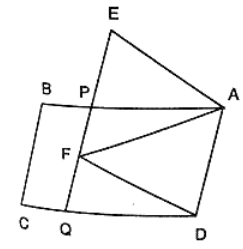Solution:

Given that, ABCD and AEFD are two parallelograms

(i) In triangles, EPA and FQD

∠PEA = ∠QFD         [corresponding angles]

∠EPA=∠FQD           [corresponding angles]

PA = QD                   [opposite sides of parallelogram]

Then, ΔEPA ≅ ΔFQD           [By AAS condition]

Therefore, EP = FQ               [C.P.C.T]

(ii) Since triangles, PEA and QFD stand on equal bases PE and FQ lies between the same parallels EQ and AD

Therefore, ar(ΔPEA) = ar(ΔQFD)  ... (1)

Since, triangles PEA and PFD stand on the same base PF and between same parallels PF and AD

Therefore, ar(ΔPFA) = ar(ΔPFD) .... (2)

Divide the equation 1 by equation 2

ar(ΔPEA) ar(ΔPFA) = ar(ΔQFD) ar(ΔPFD)

(iii) From part (i),

ΔEPA ≅ ΔFQD

Then, ar(ΔPEA) =  ar(ΔQFD).

Question: 27

In figure, ABCD is a parallelogram. O is any point on AC. PQ ∥  AB and LM ∥ AD. Prove that: ar(∥ gm DLOP) = ar(∥gm BMOQ).Solution:

Since a diagonal of a parallelogram divides it into two triangles of equal area

⇒ ar(ΔAPO) + ar(∥gm DLOP) + ar(ΔOLC)

⇒ ar(ΔAOM) + ar(∥gm BMOQ) + ar(ΔOQC)             ... (1)

Since AO and Oc are diagonals of parallelograms AMOP and OQCL respectively.

∴ ar(ΔAPO) = ar(ΔAMO)                .... (2)

And ar(ΔOLC) = ar(ΔOQC)              .... (3)

Subtracting 2 and 3 from 1, we get

ar(∥gm DLOP) = ar(∥gm BMOQ).

Question: 28

In a triangle ABC, if L and M are points on AB and AC respectively such that LM ∥ BC. Prove that:

(i) ar(ΔLCM) = ar(ΔLBM)

(ii) ar(ΔLBC) = ar(ΔMBC)

(iii) ar(ΔABM) = ar(ΔACL)

(iv) ar(ΔLOB) = ar(ΔMOC)

Solution:

(i) Clearly triangles LMB and LMC are on the same base LM and between the same parallels LM and BC.

∴ ar(ΔLMB) = ar(ΔLMC)               ... (1)

(ii) We observe that triangles LBC and MBC are on the same base BC and between same parallels LM and BC.

∴ ar(ΔLBC) = ar(ΔMBC)          ... (2)

(iii) We have,

ar(ΔLMB) = ar(ΔLMC)            [From 1]

⇒ ar(ΔALM) + ar(ΔLMB) = ar(ΔALM) + ar(ΔLMC)

⇒ ar(ΔABM) = ar(ΔACL)

(iv) we have,

ar(ΔLBC) = ar(ΔMBC)             [From 1]

⇒ ar(ΔLBC) − ar(ΔBOC) = ar(ΔMBC) − ar(ΔBOC)

⇒ ar(ΔLOB) = ar(ΔMOC).

Question: 29

In figure, D and E are two points on BC such that BD = DE = EC. Show that ar(ΔABD) = ar(ΔADE) = ar(ΔAEC).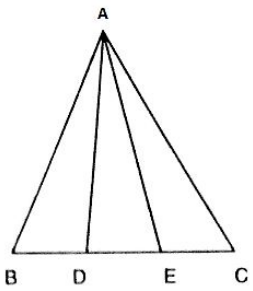Solution:

Draw a line l through A parallel to BC.

Given that, BD = DE = EC

We observed that the triangles ABD and AEC are on the equal bases and between the same parallels l and BC. Therefore, their areas are equal.

Hence, ar(ΔABD) = ar(ΔADE) = ar(ΔAEC).

Question: 30

In figure, ABC is a right angled triangle at A, BCED, ACFG and ABMN are squares on the sides BC, CA and AB respectively. Line segment AX ⊥ DE meets BC at Y. Show that

(i) ΔMBC ≅ ΔABD

(ii) ar(BYXD) = 2ar(ΔMBC)

(iii) ar(BYXD) = ar(ABMN)

(iv) ΔFCB ≅ ΔACE

(v) ar(CYXE) = 2ar(ΔFCB)

(vi) ar(CYXE) = ar(ACFG)

(vii) ar(BCED) = ar(ABMN) + ar(ACFG)Solution:

(i) In ΔMBC and ΔABD, we have

MB = AB

BC = BD

And ∠MBC = ∠ABD        [since, ∠MBC and ∠ABC are obtained by adding ∠ABC to a right angle.]

So, by SAS congruence criterion, we have

ΔMBC ≅ ΔABD

⇒ ar(ΔMBC) = ar(ΔABD)    ⋅⋅⋅⋅⋅ (1)

(ii) Clearly, triangle ABC and rectangle BYXD are on the same base BD and between the same parallels AX and BD.

∴ ar(ΔABD) = (1/2) ar(rect BYXD)

⇒ ar (rect BYXD) = 2ar(ΔABD)

⇒ ar (rect BYXD) = 2ar(ΔMBC)  ⋅⋅⋅⋅ (2)           [From  equ .1]

(iii) Since triangles MBC and square MBAN are on the same base Mb and between the same parallels MB and NC.

∴ 2ar(ΔMBC) = ar(MBAN)  ⋅⋅⋅⋅⋅ (3)

From equ. 2 and 3, we have

ar(sq. MBAN) = ar(rect BYXD)

(iv) In triangles FCB and ACE, we have

FC = AC

CB = CE

And, ∠FCB = ∠ACE    [since, ∠FCB and ∠ACE are obtained by adding ∠ACB to a right angle.]

So, by SAS congruence criterion, we have

ΔFCB ≅ ΔACE

(v) We have,

ΔFCB ≅ ΔACE

⇒ ar(ΔFCB) = ar(ΔACE)

Clearly, triangle ACE and rectangle CYXE are on the same base CE and between same parallels CE and AX.

∴ 2ar(ΔACE) = ar(CYXE)

⇒ 2ar(ΔFCB) = ar(ΔCYXE) ⋅⋅⋅ (4)

(vi) Clearly, triangle FCb and rectangle FCAG are on the same base FC and between the same parallels FC and BG.

∴ 2ar(ΔFCB) = ar(FCAG)  ⋅⋅⋅⋅ (5)

From 4 and 5, we get

ar(CYXE) = ar(ACFG)

(vii) Applying Pythagoras theorem in triangle ACB, we have

BC2 = AB2 + AC2

⇒ BC × BD = AB × MB + AC × FC

⇒ ar(BCED) = ar(ABMN) + ar(ACFG)
```### Course Features

• 728 Video Lectures
• Revision Notes
• Previous Year Papers
• Mind Map
• Study Planner
• NCERT Solutions
• Discussion Forum
• Test paper with Video Solution